Author: Oscar Cronquist Article last updated on August 11, 2019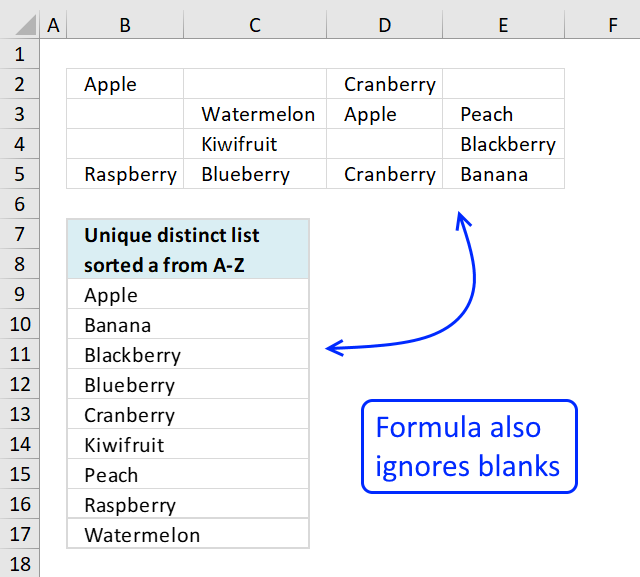This is an answer to a question in this blog post: Extract a unique distinct list sorted from A-Z from range in excel

Question: This is exactly what I've been looking for... almost. It breaks if there are blank cells in the named range. Is there a way to get this to work if there are blanks in the range?

Answer: There are two things you can consider.

(1) Fill the blanks with some text

1. Select the range
2. Press F5
3. Press with left mouse button on "Special..."
4. Press with left mouse button on "Blanks"
5. Press with left mouse button on OK!
6. Type A
7. Press Ctrl + Enter

All blanks are filled with the letter A. Remember that your new unique distinct list will contain A.

or

(2)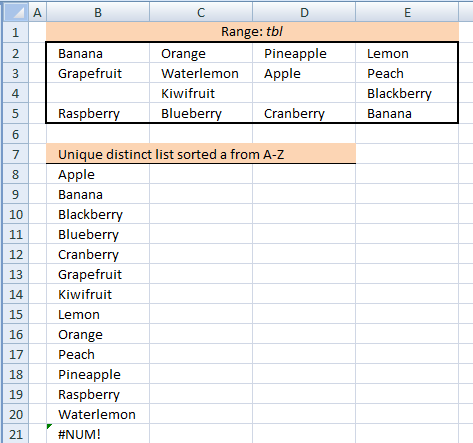Array formula in B8:

=INDEX(\$B\$2:\$E\$5, SMALL(IF(SMALL(IF(COUNTIF(\$B\$7:B9, \$B\$2:\$E\$5)+ISBLANK(\$B\$2:\$E\$5)=0, COUNTIF(\$B\$2:\$E\$5, "<"&\$B\$2:\$E\$5)+1, ""), 1)=IF(ISBLANK(\$B\$2:\$E\$5), "", COUNTIF(\$B\$2:\$E\$5, "<"&\$B\$2:\$E\$5)+1), ROW(\$B\$2:\$E\$5)-MIN(ROW(\$B\$2:\$E\$5))+1), 1), MATCH(MIN(IF(COUNTIF(\$B\$7:B9, \$B\$2:\$E\$5)+ISBLANK(\$B\$2:\$E\$5)>0, "", COUNTIF(\$B\$2:\$E\$5, "<"&\$B\$2:\$E\$5)+1)), INDEX(IF(ISBLANK(\$B\$2:\$E\$5), "", COUNTIF(\$B\$2:\$E\$5, "<"&\$B\$2:\$E\$5)+1), SMALL(IF(SMALL(IF(COUNTIF(\$B\$7:B9, \$B\$2:\$E\$5)+ISBLANK(\$B\$2:\$E\$5)=0, COUNTIF(\$B\$2:\$E\$5, "<"&\$B\$2:\$E\$5)+1, ""), 1)=IF(ISBLANK(\$B\$2:\$E\$5), "", COUNTIF(\$B\$2:\$E\$5, "<"&\$B\$2:\$E\$5)+1), ROW(\$B\$2:\$E\$5)-MIN(ROW(\$B\$2:\$E\$5))+1), 1), , 1), 0), 1)

To enter an array formula, type the formula in a cell then press and hold CTRL + SHIFT simultaneously, now press Enter once. Release all keys.

The formula bar now shows the formula with a beginning and ending curly bracket telling you that you entered the formula successfully. Don't enter the curly brackets yourself.

Then copy cell B8 and paste it down as far as necessary.

How to implement array formula to your workbook
If your list starts at, for example, F3. Change \$B\$7:B7 in the above formulas to F2:\$F\$2.

### Explaining array formula in cell B8

#### Step 1 - Count previous values are ignored

The COUNTIF function counts cells based on a condition, however, in this case, I am using it to check that no duplicates are returned.

COUNTIF(\$B\$7:B7,\$B\$2:\$E\$5)

returns {0,0,0,0;0,0,0,0;0,0,0,0;0,0,0,0}.

The array above contains only 0's (zeros), this means that no value has yet been shown. Cell range \$B\$7:B7 expands as the formula is copied to cells below, this makes sure that all previous values are checked.

#### Step 2 - Identify blank cells

The ISBLANK function returns TRUE if cell is empty and FALSE if it contains at least one character.

ISBLANK(\$B\$2:\$E\$5)

returns {FALSE, TRUE, TRUE, TRUE; TRUE, FALSE, FALSE, FALSE; TRUE, FALSE, TRUE, FALSE; FALSE, FALSE, FALSE, FALSE}.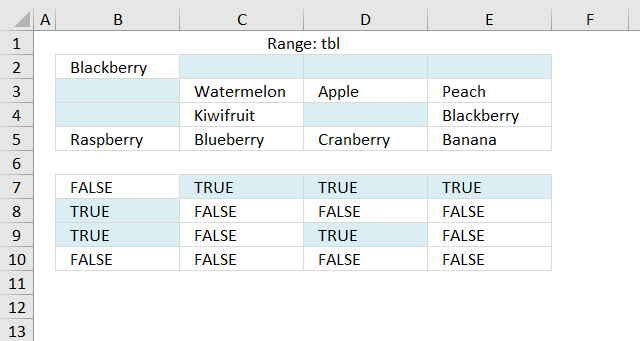The image above shows the array entered in cell range B7:E10, boolean values TRUE corresponds to the empty values in cell range B2:E5.

#### Step 3 - Add arrays and compare to zero

COUNTIF(\$B\$7:B9, \$B\$2:\$E\$5)+ISBLANK(\$B\$2:\$E\$5)=0

becomes

{0,0,0,0;0,0,0,0;0,0,0,0;0,0,0,0}+{FALSE, TRUE, TRUE, TRUE; TRUE, FALSE, FALSE, FALSE; TRUE, FALSE, TRUE, FALSE; FALSE, FALSE, FALSE, FALSE}=0

becomes

{0,1,1,1;1,0,0,0;1,0,1,0;0,0,0,0}=0

and returns

{TRUE,FALSE,FALSE,FALSE;FALSE,TRUE,TRUE,TRUE;FALSE,TRUE,FALSE,TRUE;TRUE,TRUE,TRUE,TRUE}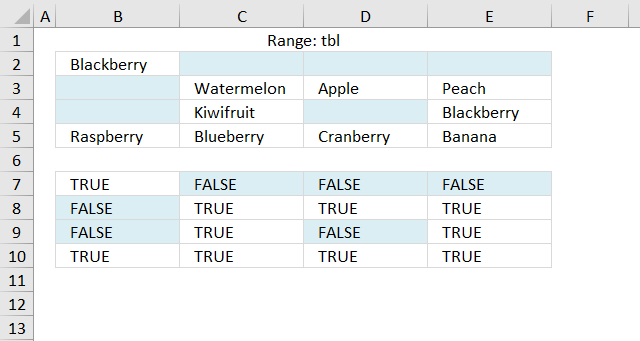The array above keeps track of blank cells and prior values.

#### Step 4 - Replace boolean values with  numbers based on their relative position if they were sorted

IF(COUNTIF(\$B\$7:B7,\$B\$2:\$E\$5)+ISBLANK(\$B\$2:\$E\$5)=0,COUNTIF(\$B\$2:\$E\$5,"<"&\$B\$2:\$E\$5)+1,"")

becomes

IF({TRUE,FALSE, FALSE,FALSE;FALSE,TRUE, TRUE,TRUE;FALSE,TRUE, FALSE,TRUE;TRUE,TRUE, TRUE,TRUE},{3,1,1,1;1,10,1,8;1,7,1,3;9,5,6,2},"")

and returns

{3,"","","";"",10,1,8;"",7,"",3;9,5,6,2}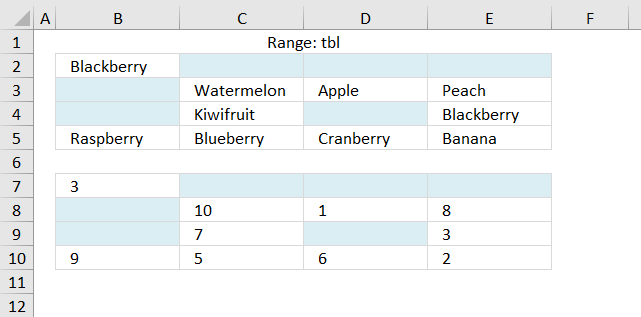#### Step 5 - Get smallest value in array

SMALL(IF(SMALL(IF(COUNTIF(\$B\$7:B9, \$B\$2:\$E\$5)+ISBLANK(\$B\$2:\$E\$5)=0, COUNTIF(\$B\$2:\$E\$5, "<"&\$B\$2:\$E\$5)+1, ""), 1)

becomes

SMALL({3,"","","";"",10,1,8;"",7,"",3;9,5,6,2}, 1)

and returns 1.

#### Step 6 - Replace smallest value with row number

IF(SMALL(IF(COUNTIF(\$B\$7:B7, \$B\$2:\$E\$5)+ISBLANK(\$B\$2:\$E\$5)=0, COUNTIF(\$B\$2:\$E\$5, "<"&\$B\$2:\$E\$5)+1, ""), 1)=IF(ISBLANK(\$B\$2:\$E\$5), "", COUNTIF(\$B\$2:\$E\$5, "<"&\$B\$2:\$E\$5)+1), ROW(\$B\$2:\$E\$5)-MIN(ROW(\$B\$2:\$E\$5))+1)

becomes

IF(1=IF(ISBLANK(\$B\$2:\$E\$5), "", COUNTIF(\$B\$2:\$E\$5, "<"&\$B\$2:\$E\$5)+1), ROW(\$B\$2:\$E\$5)-MIN(ROW(\$B\$2:\$E\$5))+1)

becomes

IF(1={3, "", "", "";"", 10, 1, 8;"", 7, "", 3;9, 5, 6, 2}, ROW(\$B\$2:\$E\$5)-MIN(ROW(\$B\$2:\$E\$5))+1)

becomes

IF({FALSE, FALSE, FALSE, FALSE;FALSE, FALSE, TRUE, FALSE;FALSE, FALSE, FALSE, FALSE;FALSE, FALSE, FALSE, FALSE}, ROW(\$B\$2:\$E\$5)-MIN(ROW(\$B\$2:\$E\$5))+1)

becomes

=IF({FALSE, FALSE, FALSE, FALSE;FALSE, FALSE, TRUE, FALSE;FALSE, FALSE, FALSE, FALSE;FALSE, FALSE, FALSE, FALSE}, {1;2;3;4})

and returns

{FALSE, FALSE, FALSE, FALSE;FALSE, FALSE, 2, FALSE;FALSE, FALSE, FALSE, FALSE;FALSE, FALSE, FALSE, FALSE}

#### Step 7 -Get smallest value

SMALL(IF(SMALL(IF(COUNTIF(\$B\$7:B7,\$B\$2:\$E\$5)+ISBLANK(\$B\$2:\$E\$5)=0,COUNTIF(\$B\$2:\$E\$5,"<"&\$B\$2:\$E\$5)+1,""),1)=IF(ISBLANK(\$B\$2:\$E\$5),"",COUNTIF(\$B\$2:\$E\$5,"<"&\$B\$2:\$E\$5)+1),ROW(\$B\$2:\$E\$5)-MIN(ROW(\$B\$2:\$E\$5))+1),1)

becomes

SMALL({FALSE, FALSE, FALSE, FALSE;FALSE, FALSE, 2, FALSE;FALSE, FALSE, FALSE, FALSE;FALSE, FALSE, FALSE, FALSE},1)

and returns 2. This is the row number we need to get the correct value.

#### Step 8 - Extract smallest value

The following steps calculate the column number needed to get the correct value.

MIN(IF(COUNTIF(\$B\$7:B7,\$B\$2:\$E\$5)+ISBLANK(\$B\$2:\$E\$5)>0,"",COUNTIF(\$B\$2:\$E\$5,"<"&\$B\$2:\$E\$5)+1))

becomes

MIN({3,"","","";"",10,1,8;"",7,"",3;9,5,6,2})

and returns 1.

#### Step 9 - Extract array from the correct row

Get array needed in the first argument in the INDEx function.

INDEX(IF(ISBLANK(\$B\$2:\$E\$5),"",COUNTIF(\$B\$2:\$E\$5,"<"&\$B\$2:\$E\$5)+1),SMALL(IF(SMALL(IF(COUNTIF(\$B\$7:B7,\$B\$2:\$E\$5)+ISBLANK(\$B\$2:\$E\$5)=0,COUNTIF(\$B\$2:\$E\$5,"<"&\$B\$2:\$E\$5)+1,""),1)=IF(ISBLANK(\$B\$2:\$E\$5),"",COUNTIF(\$B\$2:\$E\$5,"<"&\$B\$2:\$E\$5)+1),ROW(\$B\$2:\$E\$5)-MIN(ROW(\$B\$2:\$E\$5))+1),1),,1)

becomes

INDEX({3, "", "", "";"", 10, 1, 8;"", 7, "", 3;9, 5, 6, 2},SMALL(IF(SMALL(IF(COUNTIF(\$B\$7:B7,\$B\$2:\$E\$5)+ISBLANK(\$B\$2:\$E\$5)=0,COUNTIF(\$B\$2:\$E\$5,"<"&\$B\$2:\$E\$5)+1,""),1)=IF(ISBLANK(\$B\$2:\$E\$5),"",COUNTIF(\$B\$2:\$E\$5,"<"&\$B\$2:\$E\$5)+1),ROW(\$B\$2:\$E\$5)-MIN(ROW(\$B\$2:\$E\$5))+1),1),,1)

The following calculation returns the row number, I have already shown that calculation in steps 1 to 7.

INDEX({3, "", "", "";"", 10, 1, 8;"", 7, "", 3;9, 5, 6, 2},SMALL(IF(SMALL(IF(COUNTIF(\$B\$7:B7,\$B\$2:\$E\$5)+ISBLANK(\$B\$2:\$E\$5)=0,COUNTIF(\$B\$2:\$E\$5,"<"&\$B\$2:\$E\$5)+1,""),1)=IF(ISBLANK(\$B\$2:\$E\$5),"",COUNTIF(\$B\$2:\$E\$5,"<"&\$B\$2:\$E\$5)+1),ROW(\$B\$2:\$E\$5)-MIN(ROW(\$B\$2:\$E\$5))+1),1),,1)

becomes

INDEX({3, "", "", "";"", 10, 1, 8;"", 7, "", 3;9, 5, 6, 2},2,,1)

and returns {"", 10, 1, 8}

#### Step 10 - Calculate relative column number

MATCH(MIN(IF(COUNTIF(\$B\$7:B7,\$B\$2:\$E\$5)+ISBLANK(\$B\$2:\$E\$5)>0,"",COUNTIF(\$B\$2:\$E\$5,"<"&\$B\$2:\$E\$5)+1)),INDEX(IF(ISBLANK(\$B\$2:\$E\$5),"",COUNTIF(\$B\$2:\$E\$5,"<"&\$B\$2:\$E\$5)+1),SMALL(IF(SMALL(IF(COUNTIF(\$B\$7:B7,\$B\$2:\$E\$5)+ISBLANK(\$B\$2:\$E\$5)=0,COUNTIF(\$B\$2:\$E\$5,"<"&\$B\$2:\$E\$5)+1,""),1)=IF(ISBLANK(\$B\$2:\$E\$5),"",COUNTIF(\$B\$2:\$E\$5,"<"&\$B\$2:\$E\$5)+1),ROW(\$B\$2:\$E\$5)-MIN(ROW(\$B\$2:\$E\$5))+1),1),,1),0)

becomes

MATCH(1,{"", 10, 1, 8},0)

and returns 3. This is the column number needed to get the correct value.

#### Step 11 - Get value

=INDEX(\$B\$2:\$E\$5,SMALL(IF(SMALL(IF(COUNTIF(\$B\$7:B7,\$B\$2:\$E\$5)+ISBLANK(\$B\$2:\$E\$5)=0,COUNTIF(\$B\$2:\$E\$5,"<"&\$B\$2:\$E\$5)+1,""),1)=IF(ISBLANK(\$B\$2:\$E\$5),"",COUNTIF(\$B\$2:\$E\$5,"<"&\$B\$2:\$E\$5)+1),ROW(\$B\$2:\$E\$5)-MIN(ROW(\$B\$2:\$E\$5))+1),1),MATCH(MIN(IF(COUNTIF(\$B\$7:B7,\$B\$2:\$E\$5)+ISBLANK(\$B\$2:\$E\$5)>0,"",COUNTIF(\$B\$2:\$E\$5,"<"&\$B\$2:\$E\$5)+1)),INDEX(IF(ISBLANK(\$B\$2:\$E\$5),"",COUNTIF(\$B\$2:\$E\$5,"<"&\$B\$2:\$E\$5)+1),SMALL(IF(SMALL(IF(COUNTIF(\$B\$7:B7,\$B\$2:\$E\$5)+ISBLANK(\$B\$2:\$E\$5)=0,COUNTIF(\$B\$2:\$E\$5,"<"&\$B\$2:\$E\$5)+1,""),1)=IF(ISBLANK(\$B\$2:\$E\$5),"",COUNTIF(\$B\$2:\$E\$5,"<"&\$B\$2:\$E\$5)+1),ROW(\$B\$2:\$E\$5)-MIN(ROW(\$B\$2:\$E\$5))+1),1),,1),0),1)

becomes

=INDEX(\$B\$2:\$E\$5,2,3)

and returns "Apple" in cell B8.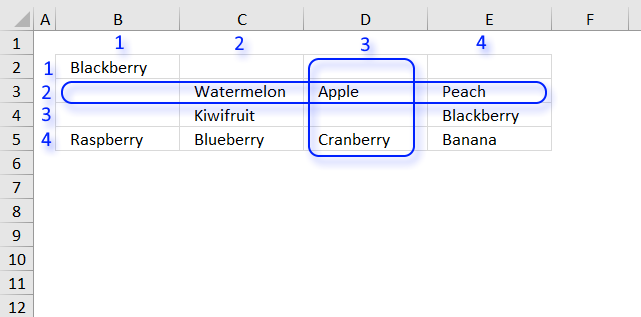Learn how to filter a multi-column and multi-row range and sort the result from A to Z:

Sort a range from A to Z [Array formula]

Question: How do I sort a range alphabetically using excel array formula? Answer: Cell range \$B\$2:\$E\$5 contains text values in random […]

Learn how to filter unique distinct values from a multi-column and multi-row cell range:

Extract unique distinct values from a multi-column cell range

Question: I have cell values spanning over several columns and I want to create a unique list from that range. […]

The following article demonstrates how to filter a unique distinct list sorted from A to Z, from a multi-column and multi-row cell range:

Extract a unique distinct list sorted from A-Z from range

The image above shows an array formula in cell B8 that extracts unique distinct values sorted alphabetically from cell range […]

How to filter duplicate values from a multi-column and multi-row cell range:

Extract duplicates from a range

The following array formula in cell B11 extracts duplicates from cell range B3:E8, only one instance of each duplicate is […]

This post shows you how to filter a multi-column and multi-row cell range based on frequency:

Sort a range based on value frequency

The formula in cell B8 extracts a list sorted based on frequency. Array formula in B8: =TEXTJOIN("", TRUE, IF(MIN(IF((MAX(IF(COUNTIF(\$B\$7:B7, \$B\$2:\$E\$5)=0, […]